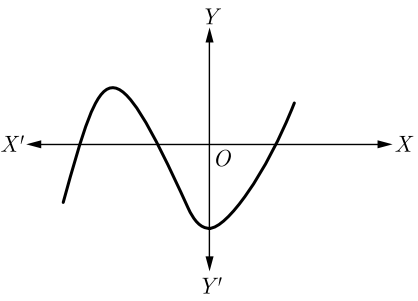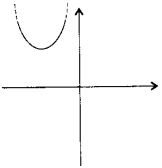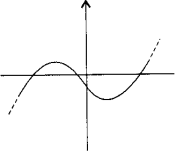Friday, October 22, 2021
Home > CBSE Class 10 > Polynomials CBSE Class 10 Maths MCQ with Answer Keys Solutions PDF DownloadPolynomials CBSE Class 10 Maths MCQ Question No 11:

If one of the zeroes of the cubic polynomial x3 + ax² + bx + c is -1, then the product of the other two zeroes is

Option A : b – a + 1

Option B : b – a – 1

Option C : a – b + 1

Option D : a – b – 1

Option A : b – a + 1

Polynomials CBSE Class 10 Maths MCQ Question No 12:

A quadratic polynomial, whose zeroes are –3 and 4, is

Option A : x2 – x + 12

Option B : x2 + x + 12

Option C : x2/2 – x/2 – 6

Option D : 2x2 + 2x + 24

Option D : 2x2 + 2x + 24

Polynomials CBSE Class 10 Maths MCQ Question No 13:

If one zero of a quadratic polynomial kx2 + 3x + k is 2, then the value of k is

Option A : 5/6

Option B : -5/6

Option C : 6/5

Option D : -6/5

Option D : -6/5

Polynomials CBSE Class 10 Maths MCQ Question No 14:

The zeroes of the quadratic polynomial x² + kx + k, k ≠ 0

Option A : cannot both be positive

Option B : cannot both be negative

Option C : are always unequal

Option D : are always equal

Option A : cannot both be positive

Polynomials CBSE Class 10 Maths MCQ Question No 15:

If one of the zeroes of a quadratic polynomial of the form x2 +ax + b is the negative of the other, then it

Option A : has no linear term and the constant term is negative

Option B : has no linear term and the constant term is positive

Option C : can have a linear term but the constant term is negative

Option D : can have a linear term but the constant term is positive

Option A : has no linear term and the constant term is negative

Polynomials CBSE Class 10 Maths MCQ Question No 16:

The graph of a polynomial is shown in figure below, then the number of its zeroes isOption A : 1

Option B : 2

Option C : 3

Option D : 4

Option C : 3

Polynomials CBSE Class 10 Maths MCQ Question No 17:

If the zeroes of the quadratic polynomial ax² + bx + c, a ≠ 0 are equal, then

Option A : c and a have opposite signs

Option B : c and b have opposite signs

Option C : c and a have the same sign

Option D : c and b have the same sign

Option C : c and a have the same sign

Polynomials CBSE Class 10 Maths MCQ Question No 18:

The quadratic polynomial, the sum of whose zeroes is -5 and their product is 6, is

Option A : x2 + 5x + 6

Option B : x2 – 5x + 6

Option C : x2 – 5x – 6

Option D : – x2 + 5x + 6

Option A : x2 + 5x + 6

Polynomials CBSE Class 10 Maths MCQ Question No 19:

Which of the following is not the graph of quadratic polynomial?

Option A :Option B :Option COption DOption D : It is not the graph of quadratic polynomial.

Polynomials CBSE Class 10 Maths MCQ Question No 20:

If one zero of the polynomial 3×2 + 8x + k is the reciprocal of the other, then the value of k is

Option A : 3

Option B : -3

Option C : 1/3

Option D : -1/3